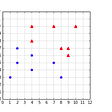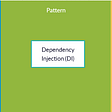# The 5 Stages of Statistical G.r.i.e.f.s.

3. “(I) can’t do this, but I can’t give up”

4. (E)at a snack

5. (F)ind out you are overcomplicating a relatively simple problem

6. (S)olve

Today, I simply want to share a humbling experience I stumbled through last week. I rode a statistical roller coaster, and it was quite the doozy.

It all started on a Monday. I was working through a simple conditional probability exercise. Here is the problem:

# Simple…right?

I’ll first explain my original answer and rationale.

Let’s start from the top. Here is how I interpreted and visualized the question:

2. The first die lands on either a 5 or a 6.

or

3. Now I ask the question: Given this first die is either sitting with 5 dots or 6 dots up, what is the probability that my second die, when added, will equal 8?

or

Here is what we know

• When I roll the second die, it has 6 equally likely outcomes: 1, 2 ,3 ,4 ,5 , or 6
• This means that ⅙ of the time, my second die will land on the complement of my 5 or 6(3 or 2) resulting in a sum of 8.

or

## Thus, my original solution:

Obviously, the answer to the question is ⅙. As I postulated, ⅙ of the time the sum of my dice will equal 8.

Me approaching the solution branch:

## The numbers disagreed.

I couldn’t be wrong. I was adamant. My argument was sound.

I decided to double down.

I proved my logic in excel:

I even created a simulation in python to prove it!

(⅙ = 0.1666)

Sooooo, my answer is correct and the solution is wrong. Right?

Nope.

# Where did I go wrong?

As it turns out, I was doomed from the start.

My visualization, excel work, and python simulation were all built on a false premise — that one dice was restricted to 5 or 6.

That’s right.

The problem tells us that both dice are thrown, and at least one die is greater than 4. It doesn’t tell us which one. Both die need to have equal probability of rolling all six numbers. After that is established, then we just apply our condition: Only consider the dice roll if at least one of them is greater than 4.

This time, instead of creating conclusions, let’s create an event space.

An event space: A theoretical space where all the possible combinations of our problem reside(like a multiverse, but for die rolls). In other words, these are all the possible outcomes our dice can find themselves in within our given constraint.

You’ll notice that four rows are crossed out(red). This is to prevent redundancy, as those specific dice rolls have already been accounted for.

Here, we see that 4 of the 20 events in the event space result in the dice summing to 8, hence 20%.

Here is another, perhaps more intuitive view:

At this point I understood why this worked theoretically, but I was still skeptical. So, I created a revamped simulation:

The most important difference to notice here: Instead of restricting die 1 to 5 or 6, which would only create an event space with 12 possible events(with 2 positive events, 5–3, 6–2), we have to allow both dice to land on any random integer between 1–6. As we see above, this expands the event space to 20 events, with 4 positive events(5–3, 6–2, 3–5, 2–6).

4/20 = .20 = 20%

Bona fide.

Questions?

--

--

--

## More from Bryan Keating

Love podcasts or audiobooks? Learn on the go with our new app.

## Expected Value of Random Variables -Explained Simply## Remembering the Tireless Ones## Support Vector Machines — Lecture series — Classifying data with a hyperplane## Weekly digest of Pedro Arantes — Goodhart’s Law, Proxy Variable, and Vector Space## What is the Shape of a Rotation Group?## The Amazing Story Behind John Nash’s Recommendation Letter## Hypothesis Testing: Understanding A/B testing## Customizing the Character UE5## What is Inversion of Control (IoC)?## Feeling bored? Me toooooo